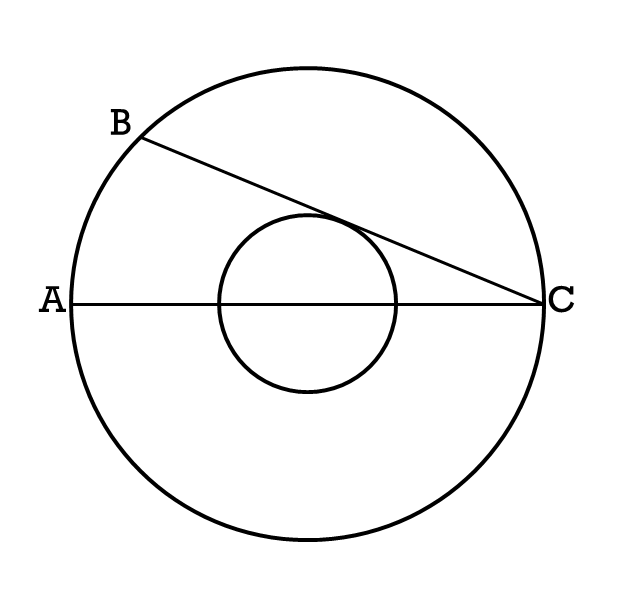# A geometry problem by Adrian Gonzalez

Geometry Level 3The ratio of the radii of two concentric circumferences is 1:3. If AC is a diameter of the largest circle, BC is a chord of the larger circle that is tangent to the small circle and AB = 18, What is the radius of the larger circle?

×